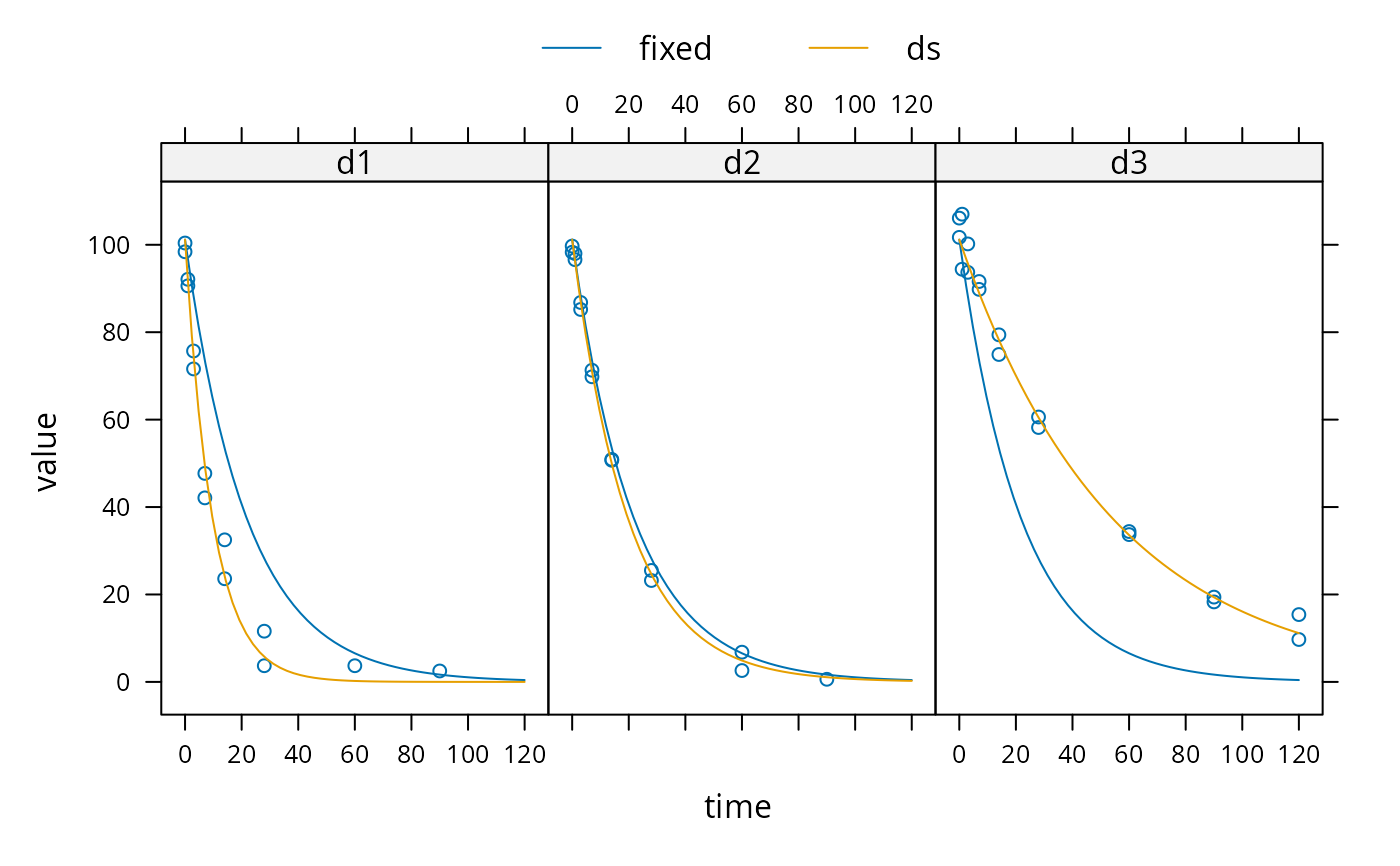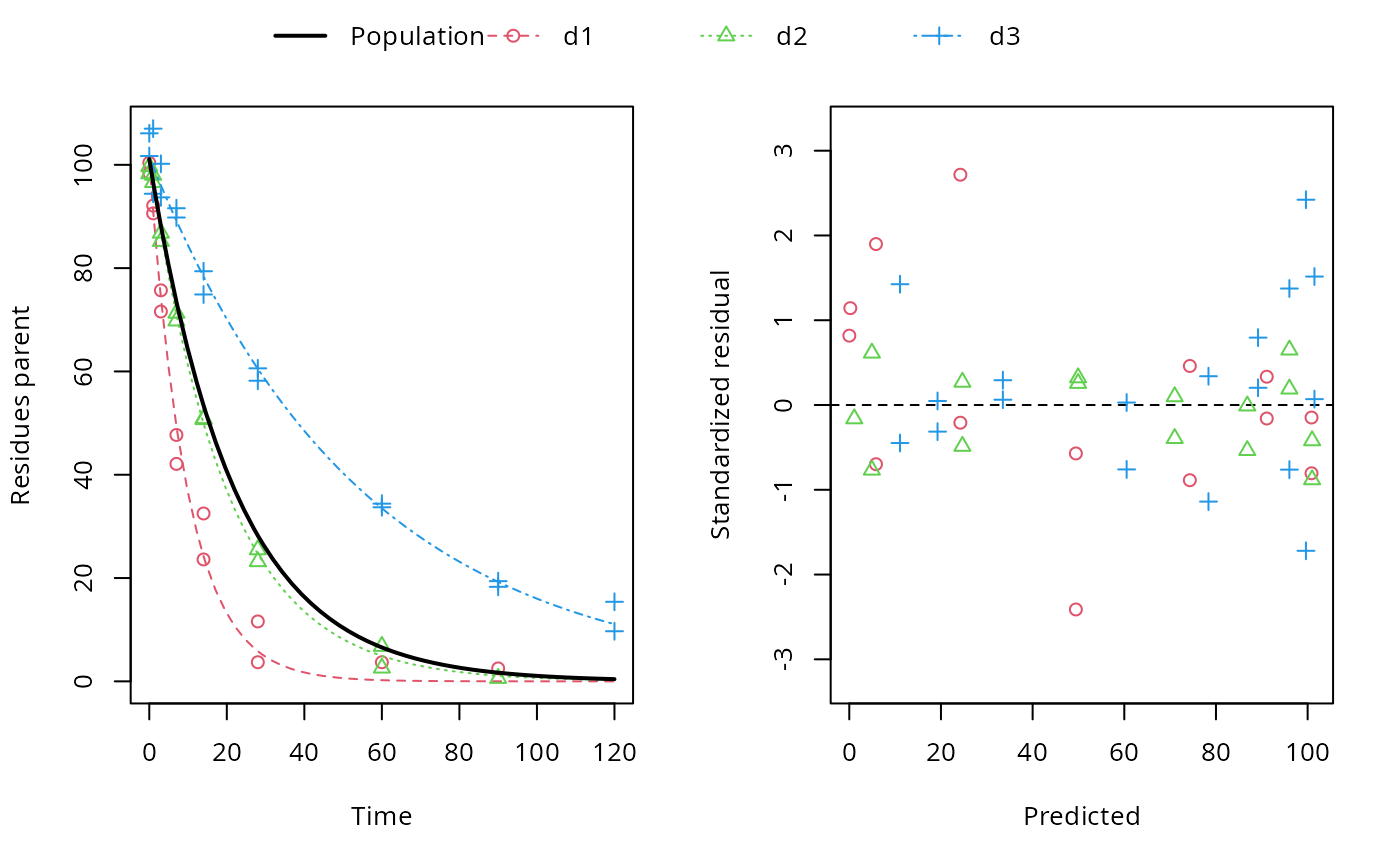These functions facilitate setting up a nonlinear mixed effects model for an mmkin row object. An mmkin row object is essentially a list of mkinfit objects that have been obtained by fitting the same model to a list of datasets. They are used internally by the nlme.mmkin() method.

nlme_function(object)

nlme_data(object)

## Arguments

object

An mmkin row object containing several fits of the same model to different datasets

## Value

A function that can be used with nlme A groupedData object

nlme.mmkin

## Examples

sampling_times = c(0, 1, 3, 7, 14, 28, 60, 90, 120)
m_SFO <- mkinmod(parent = mkinsub("SFO"))
d_SFO_1 <- mkinpredict(m_SFO,
c(k_parent = 0.1),
c(parent = 98), sampling_times)
d_SFO_1_long <- mkin_wide_to_long(d_SFO_1, time = "time")
d_SFO_2 <- mkinpredict(m_SFO,
c(k_parent = 0.05),
c(parent = 102), sampling_times)
d_SFO_2_long <- mkin_wide_to_long(d_SFO_2, time = "time")
d_SFO_3 <- mkinpredict(m_SFO,
c(k_parent = 0.02),
c(parent = 103), sampling_times)
d_SFO_3_long <- mkin_wide_to_long(d_SFO_3, time = "time")

d1 <- add_err(d_SFO_1, function(value) 3, n = 1)
d2 <- add_err(d_SFO_2, function(value) 2, n = 1)
d3 <- add_err(d_SFO_3, function(value) 4, n = 1)
ds <- c(d1 = d1, d2 = d2, d3 = d3)

f <- mmkin("SFO", ds, cores = 1, quiet = TRUE)
mean_dp <- mean_degparms(f)
grouped_data <- nlme_data(f)
nlme_f <- nlme_function(f)
# These assignments are necessary for these objects to be
# visible to nlme and augPred when evaluation is done by
# pkgdown to generate the html docs.
assign("nlme_f", nlme_f, globalenv())
assign("grouped_data", grouped_data, globalenv())

library(nlme)
m_nlme <- nlme(value ~ nlme_f(name, time, parent_0, log_k_parent_sink),
data = grouped_data,
fixed = parent_0 + log_k_parent_sink ~ 1,
random = pdDiag(parent_0 + log_k_parent_sink ~ 1),
start = mean_dp)
summary(m_nlme)
#> Nonlinear mixed-effects model fit by maximum likelihood
#>   Model: value ~ nlme_f(name, time, parent_0, log_k_parent_sink)
#>   Data: grouped_data
#>        AIC      BIC    logLik
#>   300.6824 310.2426 -145.3412
#>
#> Random effects:
#>  Formula: list(parent_0 ~ 1, log_k_parent_sink ~ 1)
#>  Level: ds
#>  Structure: Diagonal
#>         parent_0 log_k_parent_sink Residual
#> StdDev: 1.697361         0.6801209 3.666073
#>
#> Fixed effects:  parent_0 + log_k_parent_sink ~ 1
#>                       Value Std.Error DF  t-value p-value
#> parent_0          100.99378 1.3890416 46 72.70753       0
#> log_k_parent_sink  -3.07521 0.4018589 46 -7.65246       0
#>  Correlation:
#>                   prnt_0
#> log_k_parent_sink 0.027
#>
#> Standardized Within-Group Residuals:
#>        Min         Q1        Med         Q3        Max
#> -1.9942823 -0.5622565  0.1791579  0.7165038  2.0704781
#>
#> Number of Observations: 50
#> Number of Groups: 3
plot(augPred(m_nlme, level = 0:1), layout = c(3, 1))# augPred does not work on fits with more than one state
# variable
#
# The procedure is greatly simplified by the nlme.mmkin function
f_nlme <- nlme(f)
plot(f_nlme)